韩国语入门教程

(单词翻译:双击或拖选)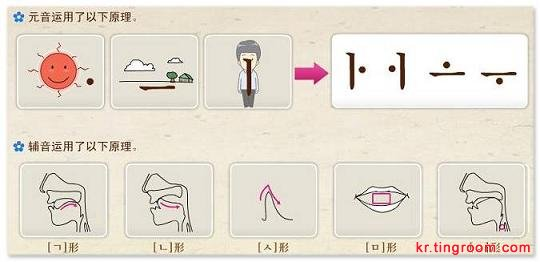?
?
? 辅音的 ?????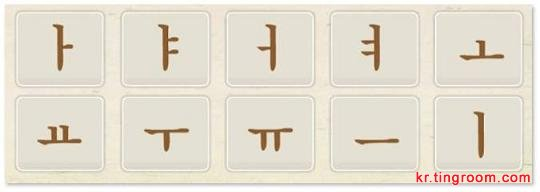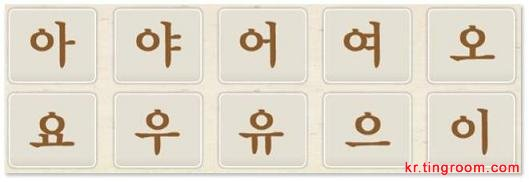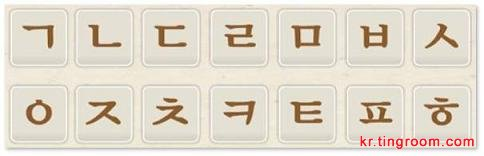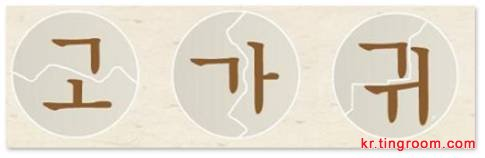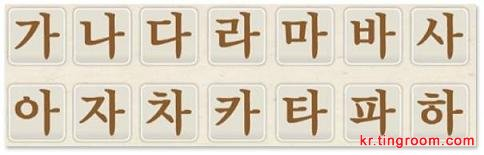??(树),
??(腿),
??(帽子),
??(公共汽车),
??(沙发),
??(棒球),
??(奶酪),
??(外套),
??(咖啡),
??(葡萄),
??(河马),
??(辣椒),
???(陶瓷),
??(鱼),
??(香皂), ??(道路), ??(方便面),
??(蝉),
?(雨),
??(洗脸),
??(邮票),
??(打针)
??(鸵鸟),
??(叉子), ??(红心)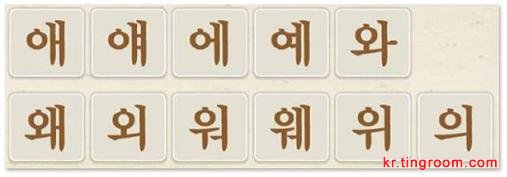??(话),
??(秋千),
??(纸币),
??(苹果),
???(为什么), ??(教会),
??(给我),
??(轨道),
??(剪刀),
??(椅子), ?(鸟),
?(脑),
?(上),
?(螃蟹),

??(婴儿),
??(毽子),
??(钟表),
??(糖果),
??(医生),
??(蝴蝶),
??(猪),
??(微笑),
??(地图),
??(猕猴桃),
??(大小),
??(画家)

??(肩膀),
??(起子),
??(同辈),
??(根),
??(爸爸),
??(写),
??(便宜),
??(咸),
??(蒸),
??(吻),
???(朱鹮),
???(打架),

???(癞蛤蟆),
??(兔子),
???(鬼),
???(布谷鸟),
???(大象)

??(话),
??(秋千),
??(纸币),
??(苹果),
???(为什么), ??(教会),
??(给我),
??(轨道),
??(剪刀),
??(椅子), ?(鸟),
?(脑),
?(上),
?(螃蟹),

??(婴儿),
??(毽子),
??(钟表),
??(糖果),
??(医生),
??(蝴蝶),
??(猪),
??(微笑),
??(地图),
??(猕猴桃),
??(大小),
??(画家)

??(肩膀),
??(起子),
??(同辈),
??(根),
??(爸爸),
??(写),
??(便宜),
??(咸), #p#分页标题#e#
??(蒸),
??(吻),
???(朱鹮),
???(打架),

???(癞蛤蟆),
??(兔子),
???(鬼),
???(布谷鸟),
???(大象)

Tag： 韩国语入门